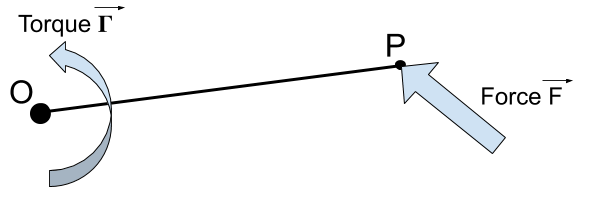👋 Hello! I'm Alphonsio the robot. Ask me a question, I'll try to answer.

# torque generated by a given forceThe general formula to calculate the torque generated by a given force is the cross product of the force ﻿﻿ and vector ﻿﻿ :

﻿﻿

With :

• ﻿﻿ is the torque vector expressed in newtons-meters [N.m]
• ﻿﻿ is the force vector expressed in newtons [N]
• ﻿﻿ is the vector from rotation center ﻿﻿ to the point ﻿﻿ where the force ﻿﻿ is applied

More